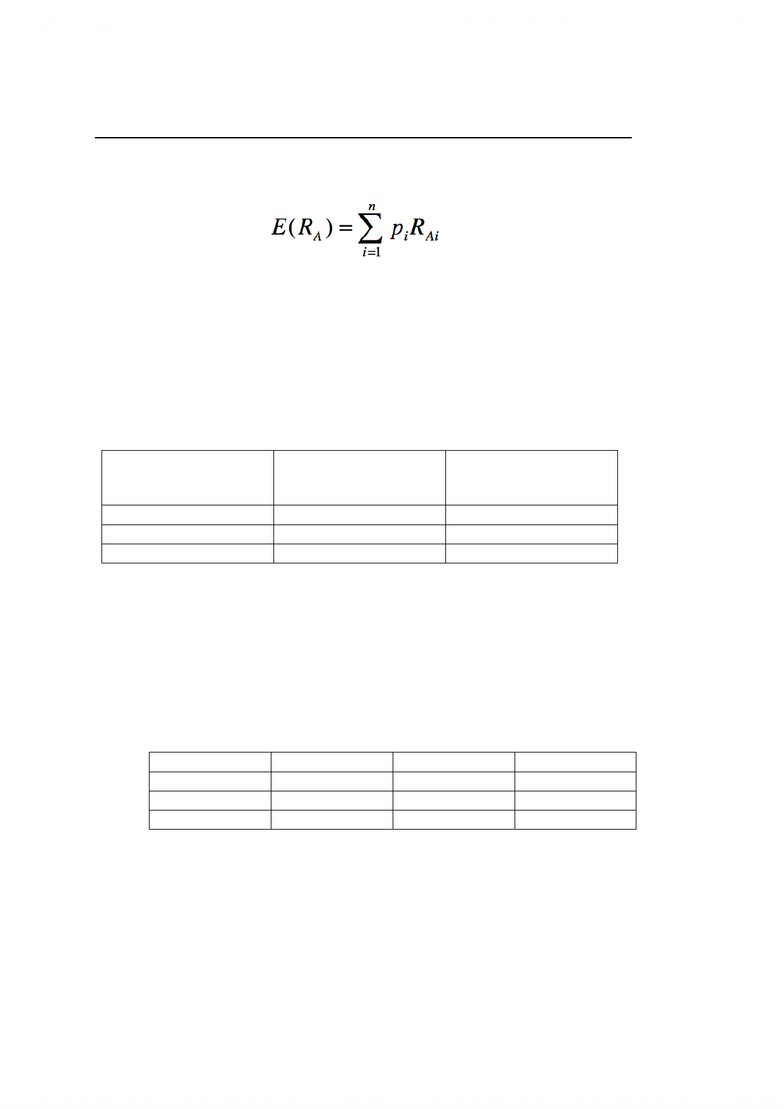Get 2 days of unlimited access
Class Notes (1,000,000)
NZ (300)
Otago (100)
(10)
Lecture

# BSNS108 Lecture Notes - Capital Asset Pricing Model, Standard Deviation

Department
Course Code
BSNS108
Professor
Helen Lu

This preview shows half of the first page. to view the full 3 pages of the document.Topic 10- Risk, Return, Portfolios, Diversification and the CAPM
Forward looking expected returns
- Expected returns depend upon possible outcomes weighted by their probabilities
Elements of the equation
E(RA)=Expected Return
pi=probability of i
RAi=rate of return
This equation appears complicated but it is actually relatively straightforward
In simple terms it is: E(RA)= Probability 1 x Return 1 + Probability 2 x Return 2 ……
Example to show this
State
Probability of state of
economy
Rate of return
Boom
0.14
0.18
Normal
0.75
0.11
Recession
0.11
-0.06
What is the future expected rate of return?
E(RA)=Probability 1 x Return 1 + Probability 2 x Return 2 + Probability 3 x Return 3
0.14 x 0.18 + 0.75 x 0.11 + 0.11 x -0.06 = 0.1011
0.1011 x 100 = 10.11
Another example to reinforce the point
Suppose there are three possible future states of the world, and you have predicted the
following returns for stocks BDA and CEC in these states. What are the expected returns
on the stocks?
State
Probability
BDA return
CEC Return
Boom
0.3
0.15
0.25
Normal
0.5
0.10
0.20
Recession
0.2
0.02
0.01
E(RBDA)=0.3 x 0.15 + 0.5 x 0.10 + 0.2 x 0.02
= 0.099
0.099 x 100 = 9.9%
E(RCEC)=0.3 x 0.25 + 0.5 x 0.20 + 0.2 x 0.01
= 0.177
0.177 x 100 = 17.7%
###### You're Reading a Preview

Unlock to view full version